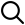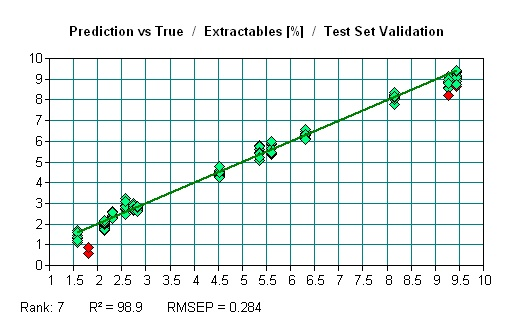QTA >> Our Services >> Development and Validation

# Development and ValidationIn order to use the QTA System for the analysis of materials, calibration algorithms must be built for the material, components, and properties of interest. For example, we need to have a soybean moisture model to analyze the moisture of a soybean sample; and a glyphosate model to analyze the concentration of glyphosate in RoundUp.

Building a calibration model requires two things: a set of calibration samples and accurate analysis data for those samples. The samples should cover the possible concentration range of the material that is going to be analyzed. If possible, it is also necessary to cover all the possible sample varieties to be analyzed in the future. For example, if the customer wants to analyze all types of corn, then the sample training set should contain feed corn, sweet corn, popcorn, etc.

The analysis data required is called primary data. Primary data is normally thought of as the traditional, wet chemical or chromatographic technique that the customer uses to analyze their materials. Most often, these directly measure the component of interest – for example, measuring viscosity using a viscometer, or measuring water content using a Karl Fischer titrator – so these are called direct methods and primary methods, and thus the data obtained is primary data.

Infrared spectroscopy as used by QTA, on the other hand, does not do direct measurements of the components and properties of interest. Rather, QTA builds a correlation of the QTA light spectra to the primary data. Therefore, QTA and infrared spectroscopy is termed as a “secondary method” since it does not analyze the material directly but rather correlates to light spectra of previously analyzed samples (the calibration training set).

To build a calibration, between 30 samples and 200 samples are required. The number of samples needed is dependent on the homogeneity of the sample, and the range of sample interest. The calibration samples are measured by QTA MIR/NIR instrument to obtain the MIR/NIR spectra. The obtained spectra and the provided primary data are used to build the calibration model. Most of QTA models are built using a PLS (partial least squares) method. The calibration is usually displayed as shown in the following graph:The above calibration graph shows the model prediction value vs. the primary data, with the primary data value on the x-axis and the value predicted by QTA on the y-axis. Two terms are used to interpret these data:

• Correlation coefficient: R2 is the square the square of the correlation coefficient. A higher Rmeans a better correlation.
• Error of prediction: Root mean square error of prediction (RMSEP) represents the standard error of the calibration model or the standard deviation of the calibration model. RMSEP is the number used to define the accuracy of the model prediction. From the normal distribution, 68% of the unknown samples analyzed will have a predicted value that is within one standard error; 95% is within twice of the standard error, and 99.7% is within 3 times of the standard error. For example, if a model with the RMSEP of 0.1%, the probability to predict a sample with the error of ±0.1% is 68%, the probability to predict a sample with the error of ±0.2% is 95%, and the probability to predict a sample with the error of ±0.3% is 99.7%.  For a given set of samples, the higher the R2 the lower the RMSEP is. However, Rdepends on the range of the concentration. With a small concentration range, a smaller R2 does not mean less accuracy.

### Validation Process:

Once the development process is complete, and the requirements for correlation coefficient and standard error are satisfied, the Validation Process begins. Validation is performed for all customers, whether they are using an industry standard calibration (such as for biodiesel analysis), or if QTA has developed custom calibrations for a material.

The validation process is an iterative process. Following development, additional samples and primary data are obtained. The samples are then analyzed using the QTA System, and the results are compared with the primary data. If the results are within 2 standard errors, then the validation is satisfied. If the results are not within 2 standard errors, then several things are investigated:

• The primary data is re-determined, to ensure its accuracy.

• If accurate, then the sample matrix for this sample may not have been included in the calibration set. Investigations will be done by the QTA team to determine whether this new sample can be added to the calibration

• The process continues until all samples, primary data and QTA results fall within the 2 standard error requirement.